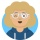Home
IT Knowledge
Inspiration
Languages
EN

# Python - convert string to double

0 points
Created by:Antonina-Trejo
237

Unfortunately, there are no doubles in Python. You can use float that behaves like a double as an alternative solution.

Quick solution:

``````number = "1.234"
result = float(number)

print(type(result))  # <class 'float'>``````

or:

``````from decimal import Decimal

number = "1.234"
result = Decimal(number)

print(type(result))  # <class 'decimal.Decimal'>``````